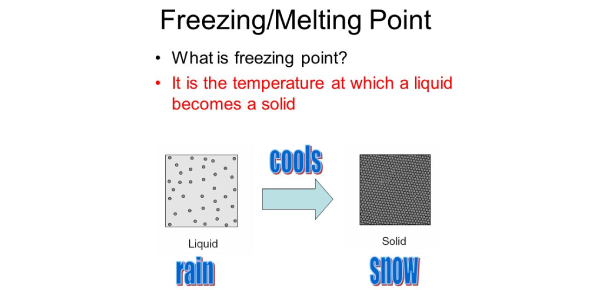# Melting And Freezing Quiz

10 Questions | Total Attempts: 1393SettingsMelting and Freezing point is one of many scientific terms used to understand the melting and freezing of the objects especially liquids. The melting and freezing points are measured by using fire and ice respectively. To know more, check out the quiz below!

• 1.
Water is very dynamic.
• A.

True

• B.

False

• C.

Sometimes

• D.

Hardly

• 2.
Which of this can water be turned into?
• A.

Ice

• B.

Liquid

• C.

Gas

• D.

All of the above

• 3.
What causes ice to melt?
• A.

Heat

• B.

Vapour

• C.

Evaporation

• D.

Transpiration

• 4.
The process of changing water to ice or liquid is called change of state of matter.
• A.

True

• B.

False

• C.

Sometimes

• D.

Unconfirmed

• 5.
What stays the same despite changes in state of matter?
• A.

Quantity

• B.

Quality

• C.

Temperature

• D.

Molecules

• 6.
How many hydrogen atoms are in water?
• A.

Two

• B.

One

• C.

Three

• D.

Five

• 7.
What is the hydrogen bonded to?
• A.

Nitrogen

• B.

Possasium

• C.

Oxygen

• D.

All of the above

• 8.
How many oxygen atoms?
• A.

Two

• B.

Three

• C.

Five

• D.

None of the above

• 9.
Which charge does the oxygen have?
• A.

Negative

• B.

Positive

• C.

Slightly positive

• D.

Slightly negative

• 10.
What is the melting point of ice?
• A.

• B.

-0°

• C.

10°

• D.

100°

Related TopicsBack to top